# Math problems

Verbal problems allow you to practice your knowledge of mathematics in problems of everyday life and school problems. Problems train understanding, translation into the mathematical language (eg equations), solve it, check the accuracy and solution discussion.
Choose a topic you want to calculate and improve in.

### From our database of math problems we offer:

• SimilarityAre two right triangles similar to each other if the first one has a acute angle 70° and second one has acute angle 20°?
• Charles Bridge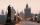Charles Bridge was 15 times older than Frantův bridge from 1900 in 1931. How old is the Charles Bridge?
• Bus linesBuses connections are started from bus stop on its regular circuit as follows: No. 27 bus every 27 minutes and No.18 every half hour. What time started this two bus lines run if the bus stop met at 10:15 am.?
• Unknown number 11That number increased by three equals three times itself?
• SimplifySimplify expression - which expression is equivalent to: 3(m + 2) − 4(2m − 9)
• Hockey teams goals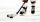Hockey teams fired 200 goals. The second team 13 less than first team, third 16 less than the first and fourth tean 19 goals less than first. How many goals fired each team?
• Evening shiftWhile working the evening shift, Officer K took 8 hours to complete a task at his work station and Officer M took 10 hours to complete the same task at his work station. How many hours would it take Officer K and Officer M to complete the same task working
• Granules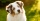Katy had two dogs. The first one ate a pack of granules a week and the other two times longer. Katka bought them two bags of granules. How long does it last for both dogs?
• A candleA candle shop sells scented candles for \$16 each and unscented candles for \$10 each. The shop sells 28 candles today and makes \$400. a. Write a system of linear equations that represents the situation. b. Solve the system to answer the questions: How m
• Isosceles triangle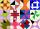Calculate the perimeter of isosceles triangle with arm length 26 cm and base length of 21 cm.
• Addition of Roman numbersAdded together and write as decimal number: LXVII + MLXIV
• BonbonsCreate a mixture of 50 kg of candy on price 700Kč. Candies has prices: 820Kč, 660Kč and 580Kč. Use cross rule.
• Right triangleLegs of right are in ratio a:b = 6:8. Hypotenuse has a length of 61 cm. Calculate the perimeter and area of the triangle.
• Speed of sound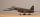The average speed of sound is 330 meters per second. Estimate how long it will hear the church bell 1 km away. Calculate the distance from what would hear sound after 10 seconds.
• Internal anglesThe ABCD is an isosceles trapezoid, which holds: |AB| = 2 |BC| = 2 |CD| = 2 |DA|: On its side BC is a K point such that |BK| = 2 |KC|, on its side CD is the point L such that |CL| = 2 |LD|, and on its side DA the point M is such that | DM | = 2 |MA|. Dete
• Cylinder-shaped vase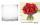If the cylinder-shaped vase is filled with water up to 35 cm, it contains 1 liter of water. How much water will it contain if it is filled to a height of 45cm?
• The perimeter 2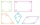The perimeter of the quadrilateral a = 1m b = 14/5m c = 2 3/10m d = 1 4/5m?
• GroupGroup of kids wanted to ride. When the children were divided into groups of 3 children 1 remain. When divided into groups of 4 children 1 remain. When divided into groups of 6 children 1 missed. After divided to groups of 5 children its OK. How many are t
• If one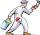If one litre of pant covers an area of 5 m2 how much paint is needed to cover: a) rectangular swimming pool With dimensions 4m x 3m x 2.5m (the Inside walls and the floor only) b) the Inside walls and floor of a cylindrical reservoir with diameter 3m and
• Proof PTCan you easy prove Pythagoras theorem using Euclidean theorems? If so, do it.